Question

# A 1150 kg car is on a 8.70 hill. Using X-Y axes tilted down the plane,...

A 1150 kg car is on a 8.70 hill. Using X-Y axes tilted down the plane, what is the x and y component of the normal force?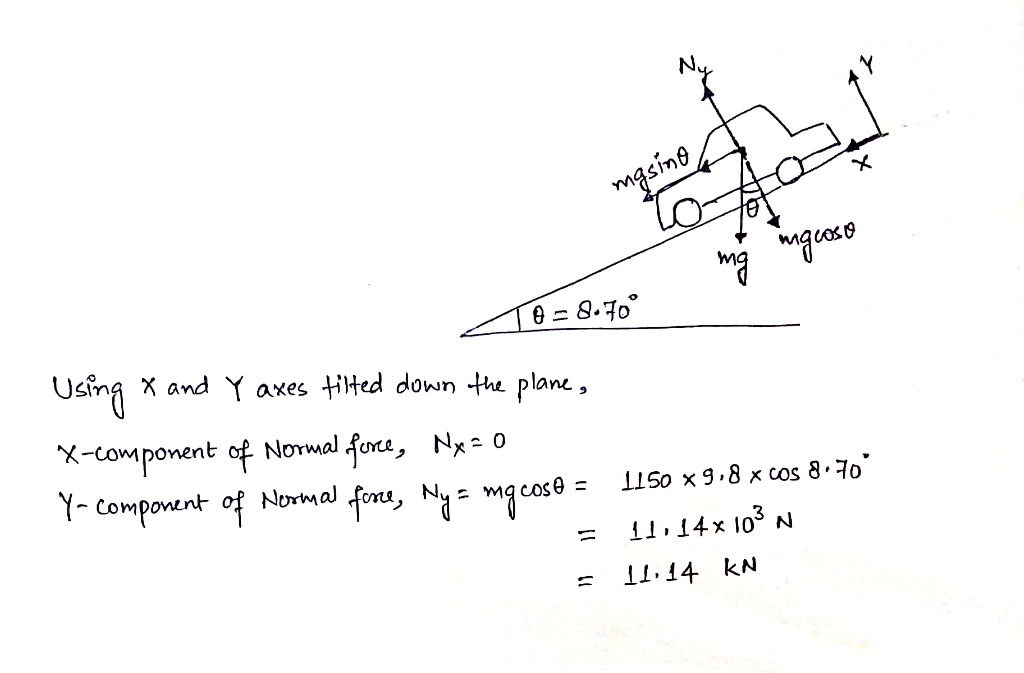#### Earn Coins

Coins can be redeemed for fabulous gifts.

Similar Homework Help Questions
• ### Problem # 4 The 3000 kg cable car shown in figure descends a 200 m-high hill....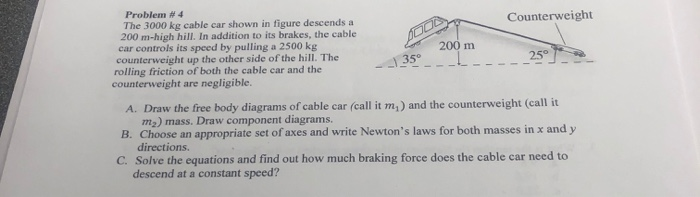Problem # 4 The 3000 kg cable car shown in figure descends a 200 m-high hill. In addition to its brakes, the cable car controls its speed by pulling a 2500 kg counterweight up the other side of the hill. The rolling friction of both the cable car and the counterweight are negligible. Counterweight 200 m 25° 35 A. Draw the free body diagrams of cable car (call it m,) and the counterweight (call it m2) mass. Draw component diagrams....

• ### The block has mass 10.0 kg and lies on a fixed smooth frictionless plane tilted at...

The block has mass 10.0 kg and lies on a fixed smooth frictionless plane tilted at an angle 25.0 degree to the horizontal. (a) Draw the free body diagram. (b) Find the magnitude of the normal force. (c) Determine the acceleration of the block as it slides down the plane. (d) If the block starts from rest 12.0m up the plane from its base, what will be the block’s speed when it reaches the bottom of the incline? (Explain in...

• ### A 1000 kg car slowly descends 20 meters down a hill covered with ice (no friction)....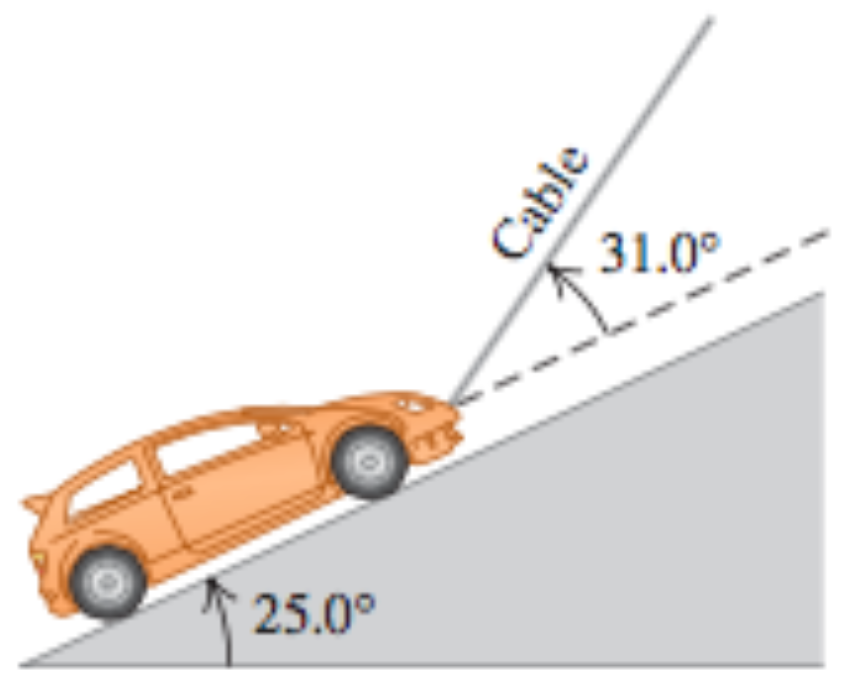A 1000 kg car slowly descends 20 meters down a hill covered with ice (no friction). See the figure. (a) How much force tension does the cable do? (b) How much work does the weight of the car do? (c) How much work does cable tension do? (d) What is the total work on the car? Cable 25.0°

• ### A sports car of mass 950 kg (including the crosses the rounded top of a hill...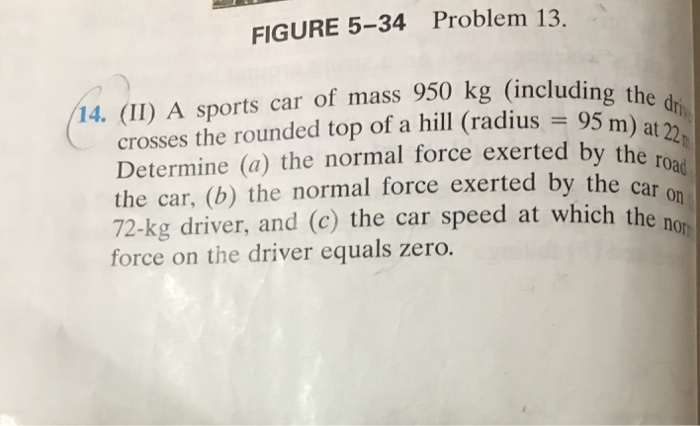A sports car of mass 950 kg (including the crosses the rounded top of a hill (radius = 95 m) at 22 Determine the normal force exerted by the road the car, the normal force exerted by the car on 72-kg driver, and the car speed at which the force on the driver equals zero.

• ### A 1100 kg car traveling at 20 m/s goes over a hill. At the top, the...

A 1100 kg car traveling at 20 m/s goes over a hill. At the top, the hill provides an upward normal force of 5600 N. (a) Assuming the hill has a circular profile, find the radius of curvature of the hill. (b) What is the maximum speed the car can go over the hill without ramping the car off the ground?

• ### 3. An 800 kg car travels over a hill having the shape of a parabola. If...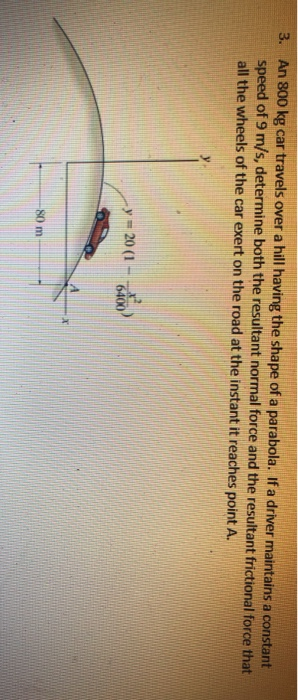3. An 800 kg car travels over a hill having the shape of a parabola. If a driver maintains a constant speed of 9 m/s, determine both the resultant normal force and the resultant frictional force that all the wheels of the car exert on the road at the instant it reaches point A. y = 20 (1 - 6400

• ### An elliptical loop of axes of length a and b, centered at origin and lying in the y − z plane car...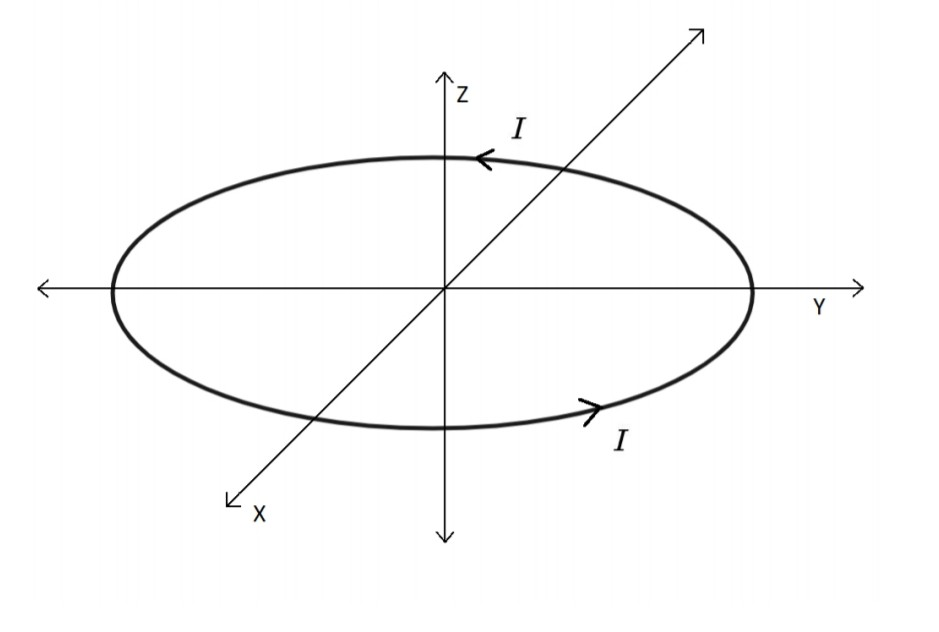An elliptical loop of axes of length a and b, centered at origin and lying in the y − z plane carries current I. The major axis of the ellipse is the ˆy. 1. What component of A is non-zero in the x − y plane? [A qualitative argument will sufce] 2. Find an expression for B in terms of th non-zero components of A. 3. What is the direction of B along i) the positive x axis and ii)...

• ### An 1100 kg car is shifted into neutral and coasts down a 10 meter hill and...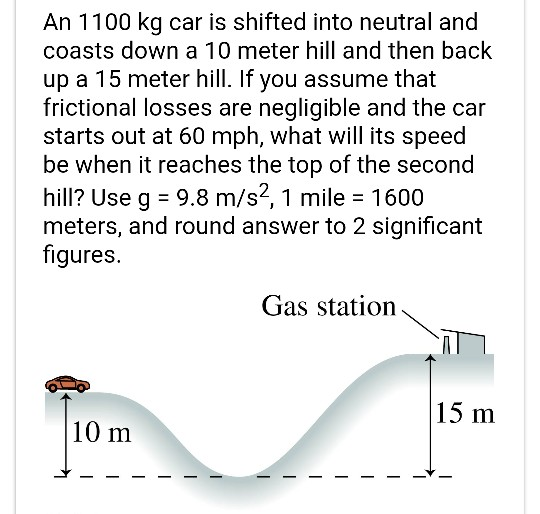An 1100 kg car is shifted into neutral and coasts down a 10 meter hill and then back up a 15 meter hill. If you assume that frictional losses are negligible and the car starts out at 60 mph, what will its speed be when it reaches the top of the second hill? Use g 9.8 m/s2, 1 mile 1600 meters, and round answer to 2 significant figures. - Gas station 15 m 10 m

• ### A135° 1. 25° Problem #4 Counterweight The 3000 kg cable car shown in figure descends a...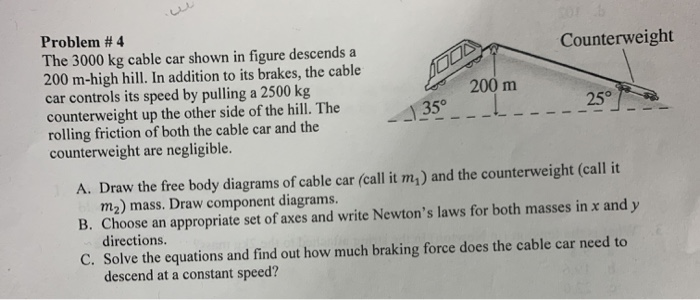A135° 1. 25° Problem #4 Counterweight The 3000 kg cable car shown in figure descends a 200 m-high hill. In addition to its brakes, the cable car controls its speed by pulling a 2500 kg 200 m counterweight up the other side of the hill. The rolling friction of both the cable car and the counterweight are negligible. A. Draw the free body diagrams of cable car (call it m ) and the counterweight (call it m2) mass. Draw component...

• ### In the figure, a car is driven at constant speed over a circular hill and then...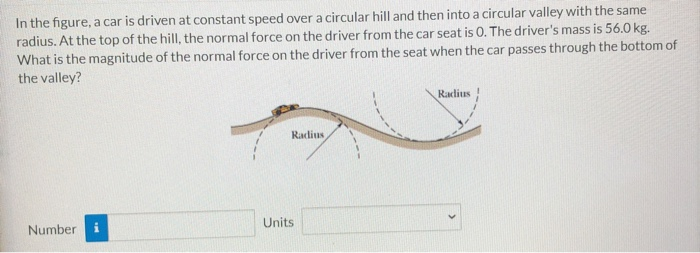In the figure, a car is driven at constant speed over a circular hill and then into a circular valley with the same radius. At the top of the hill, the normal force on the driver from the car seat is 0. The driver's mass is 56.0 kg. What is the magnitude of the normal force on the driver from the seat when the car passes through the bottom of the valley? Raclius Raclius Units Number